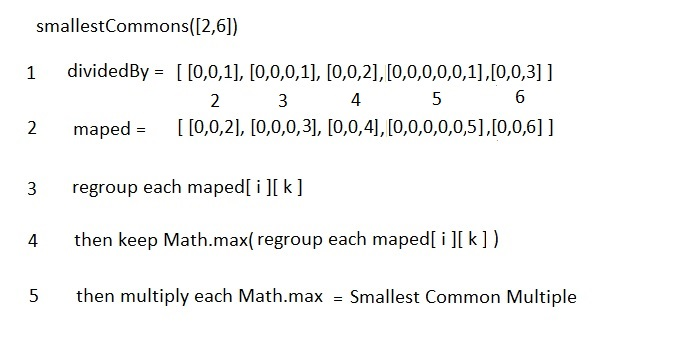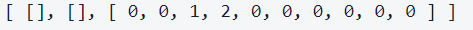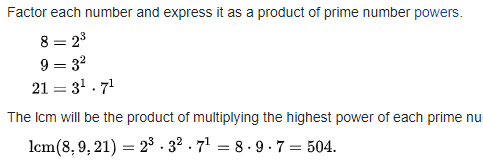# Smallest Common Multiple: infinit loop

Hello,
Smallest Common Multiple
This is what I would like to do:Whith this code , with the first loop in comment and with only one number passed in argument that works.:

``````function smallestCommons(n) {
//let max = Math.max(...arr);
//let min = Math.min(...arr);
//console.log(min)
const dividedBy = [[],[]]
//for (let n = min; n <= max; n++) {
/* I don't want to iterate over all the numbers so I'm only looking for prime numbers like in the previous exercise*/
//________________________________________________________________
let maxMultiple = Math.floor(Math.sqrt(n));
let numbers = [];
for (let l = 2; l <= n; l++) {
numbers.push(l);
}
for (let i = 2; i <= maxMultiple; i++) {
for (let j = Math.pow(i, 2) ; j <= n; j += i) {
if ( j !== false) {
numbers[j - 2] = false;
}
}
}
//console.log(numbers)
let primes = numbers.filter(item => Number.isInteger(item));
//_________________________________________________________________
primes.unshift(0,0)
console.log(primes)
let compte = 0;
dividedBy.push([0,0]);
console.log(dividedBy)
for (let k = 2; k <= primes.length; k++) {  //for each number n in the first for loop, I decompose over each prime number.

if (n % primes[k] == 0) {
let n2 = n / primes[k];
compte += 1
n = n2;
k--;
}else{
dividedBy[dividedBy.length-1].push(compte)
compte = 0
}

}
console.log(dividedBy)
//}
}
smallestCommons();
``````

For `smallestCommons()` the console return:Great!!
But when I add the first for loop, with `smallestCommons([9,18])` there is a infinity loop:

``````function smallestCommons(arr) {
let max = Math.max(...arr);
let min = Math.min(...arr);
console.log(min)
const dividedBy = [[],[]]
for (let n = min; n <= max; n++) {
/* I don't want to iterate over all the numbers so I'm only looking for prime numbers like in the previous exercise*/
//____________________________________________________________________
let maxMultiple = Math.floor(Math.sqrt(n));
let numbers = [];
for (let l = 2; l <= n; l++) {
numbers.push(l);
}
for (let i = 2; i <= maxMultiple; i++) {
for (let j = Math.pow(i, 2) ; j <= n; j += i) {
if ( j !== false) {
numbers[j - 2] = false;
}
}
}
//console.log(numbers)
let primes = numbers.filter(item => Number.isInteger(item));
//__________________________________________________________________
primes.unshift(0,0)
console.log(primes)
let compte = 0;
dividedBy.push([0,0]);
console.log(dividedBy)
for (let k = 2; k <= primes.length; k++) {  //for each number n in the first for loop, I decompose over each prime number.

if (n % primes[k] == 0) {
let n2 = n / primes[k];
compte += 1
n = n2;
k--;
}else{
dividedBy[dividedBy.length-1].push(compte)
compte = 0
}

}
console.log(dividedBy)
}
}
smallestCommons([9,18]);
``````

I need helpI honestly don’t understand the algorithm from this series of steps. Can you expand upon what you mean?

I’m not certain that this algorithm is mathematically sound. Do you have some reference material for this approach?

Using prime factorizationAh, your description of the algorithm is not a valid prime factorization. The prime factorization uses powers of primes, not multiples of primes.

Also, you should only make your array of primes once.

To try to find what is causing infinite loop, you may take a look at loops one-by-one. Double check the stopping condition, and if variables are changed in such way, that loop will end, check also if perhaps the variables aren’t changed somewhere else.

Yeah, it does look like the iteration variable is modified inside of the iteration, but the bigger problem is that the algorithm as coded is not mathematically correct. Not a lot of benefit in fixing an implementation that can’t work.

1 Like

I made a mistake in the picture with the number 6 but that works:

That mistake makes a huge difference. You aren’t really implementing this algorithm as described with your complex loop though.

Sieving with a mixed array (booleans and numbers) still is less efficient than only using a boolean array. Dynamically allocating that numbers array is still slow.

In any case, you will actually need all primes up to `max` for this algorithm to work.

You should not generate primes inside of this loop. That is a lot of repeated/wasted effort.

You should not modify `n` inside of this loop… In the best case, manual adjustment of the iteration variable is confusing. In the worst case, it is going to break your code.

I would actually step back and empty this function out and start with comments

``````function smallestCommons(arr) {
// 0 - Compute primes

// 1 - Prime factorization of each num in range

// 2 - Find maximum power of each prime

// 3 - Compute smallest common multiple
let scm;

// 4 - Return result
return scm;
}
``````

I would then add some more comments about how to do each part before starting to add code.

Yes I know because I’m blocked so I not finished.

Sorry Jeremy, I must leave now.
I will read your post in detail tomorrow.
See you tomorrow.
Enjoy afternoon.1 Like

Hello,
I took some holidays.
I resume the exercice this week and that works``````function smallestCommons(arr) {
let max = Math.max(...arr);
let min = Math.min(...arr);
const dividedByIndex = [, ];
//  0 - I'm looking for the prime of n.(Compute primes)______________________________
let isPrime = Array(max + 1).fill(true);
// 0 and 1 are not prime
isPrime = false;
isPrime = false;
for (let i = 2; i <= Math.sqrt(max); i++) {
if (isPrime[i]) {
// if i has not been marked false -- it is a prime
for (let j = i * i; j <= max; j += i)
isPrime[j] = false;
}
}
console.log('the prime numbers less than n : ' + isPrime)

for (let n = min; n <= max; n++) {
//console.log('le chiffre n : ' + n);
let count = 0;
dividedByIndex.push([0,0]); // I added two zero in order to make match count with the index egal to prime[k]

// 1 - Prime factorization of each num in range._____________________________

for (let k = 2; k < isPrime.length; k++) {
//for each number n in the first for loop, I decompose over each prime number.
let n2 = n;
if (isPrime[k]) {
while (n2 % k == 0) {
n2 = n2 / k;
count++;
}
}
dividedByIndex[dividedByIndex.length - 1].push(count);
count = 0;

} //  k loop
} // n loop
console.log('Primes factorization with count : ' + JSON.stringify(dividedByIndex));

// 2 - Find maximum power of each prime______________________________

let factorized = [];
for (let i = 0; i < dividedByIndex.length; i++) {
factorized.push([])
for (let j = 0; j < dividedByIndex[i].length; j++) {
if ( dividedByIndex[i][j] === 0 ) {
factorized[factorized.length-1].push(0) // because each number pow 0 egal 1
} else {
factorized[factorized.length-1].push(Math.pow(j, dividedByIndex[i][j]))
}
}
}
console.log(
'Product prime(index) by factorization(count) :' + JSON.stringify(factorized)
);
console.log(factorized[factorized.length - 1].length);
let factorizedByIndexGroup = [];
console.log(factorized[factorized.length - 1].length);
for (let i = 0; i < factorized[factorized.length - 1].length; i++) {
factorizedByIndexGroup.push([]);
for (let j = 0; j < factorized.length; j++) {
if (factorized[j][i] > 0) {
factorizedByIndexGroup[i].push(factorized[j][i]);
}
}
}
console.log('Power of each prime : ' + JSON.stringify(factorizedByIndexGroup));
// I delete the empty arrays:
let factorizedByIndexGroupfiltered = factorizedByIndexGroup.filter(
(x) => x.length > 0
);
console.log( factorizedByIndexGroupfiltered )
//factorizedByIndexGroupfiltered.reduce((a,b) => Math.max(...a) * Math.max(...b))
let maxPowerPrimes = factorizedByIndexGroupfiltered.map((x) => Math.max(...x));
console.log('maximum power of each primes : ' + maxPowerPrimes)

// 3 - Compute smallest common multiple (scm)__________________________

let scm = maxPowerPrimes.reduce((a,b) => a * b);
console.log(scm)
return scm
}
smallestCommons([2, 10]);
``````

I really would be interrested to have your comments in order to improve it.

In part 2 I put this line as comment because it doesn’t work. Could you please say me if it’s possible or not ?

Good job getting it to work! It is a trick approach to take.

I think the biggest issue is that your code is very complex. You have a lot of pieces to keep track of that you can ultimately simplify

``````function smallestCommons(arr) {
const [min, max] = [...arr].sort((a, b) => a - b);
const range = Array(max - min + 1).fill(0).map((_, i) => i + min);
// Find all possible prime factors
const isPrime = Array(max + 1).fill(true);
isPrime = false; isPrime = false;
for (let i = 2; i < Math.sqrt(max); i++)
if (isPrime[i])
for (let j = i*i; j <= max; j+= i)
isPrime[j] = false;
// Compute and return SCM
return isPrime
// Find highest factor of each prime
.map(
(isPrime, i) => {
return !isPrime
? 1
: range
// Find highest power of prime for each num in range
.map((num) => {
let factor = 1;
while (num % i === 0) {
factor *= i; num /= i;
}
return factor;
})
// Find highest power of prime for all num in range
.reduce((a, b) => a > b ? a : b);
}
)
// Compute SCM
.reduce((product, num) => num * product)
}

console.log(smallestCommons([2, 10]));
``````

I think it’s work trying to implement the GCD based algorithm. That is a particularly good approach.

1 Like

whaowww !I had a hard time to understand. I never imagined doing it like this, impossible for me. Should I be worried about my abilities ?

Is it really necessary to know how to do this for a “front end” developer?

Solving math focused problems like this one is a specialized skill that takes practice.

The bigger thing you should get from this challenge is breaking down a complicated problem and getting a solution that works. Once you get solutions that work, then you can practice refining them.

So you recognize that these exercises are complicated?
Which would mean that maybe I’m not so bad.

Can you answer to my question on the level required of a “front end” developer?

The challenge is complicated, but so is programming work. Don’t mistake me saying the challenge is complicated as me saying it is unreasonable.

Complicated tasks are required of all developers, front end or otherwise. The only way to get good at complicated things is to practice.

Ok,
Thank you Jeremy, see you tomorrow.

The next one was easy!!
No more than 10mn.

``````function dropElements(arr, func) {
for (let i= 0; i < arr.length; i++) {
if (func(arr[i])) {
return arr.slice(i)
}
}
return [];
}

dropElements([1, 2, 3], function(n) {return n < 3; });
``````

see you tomorrow1 Like

This topic was automatically closed 182 days after the last reply. New replies are no longer allowed.